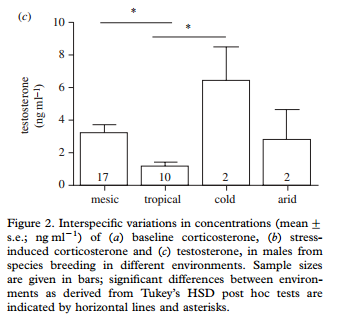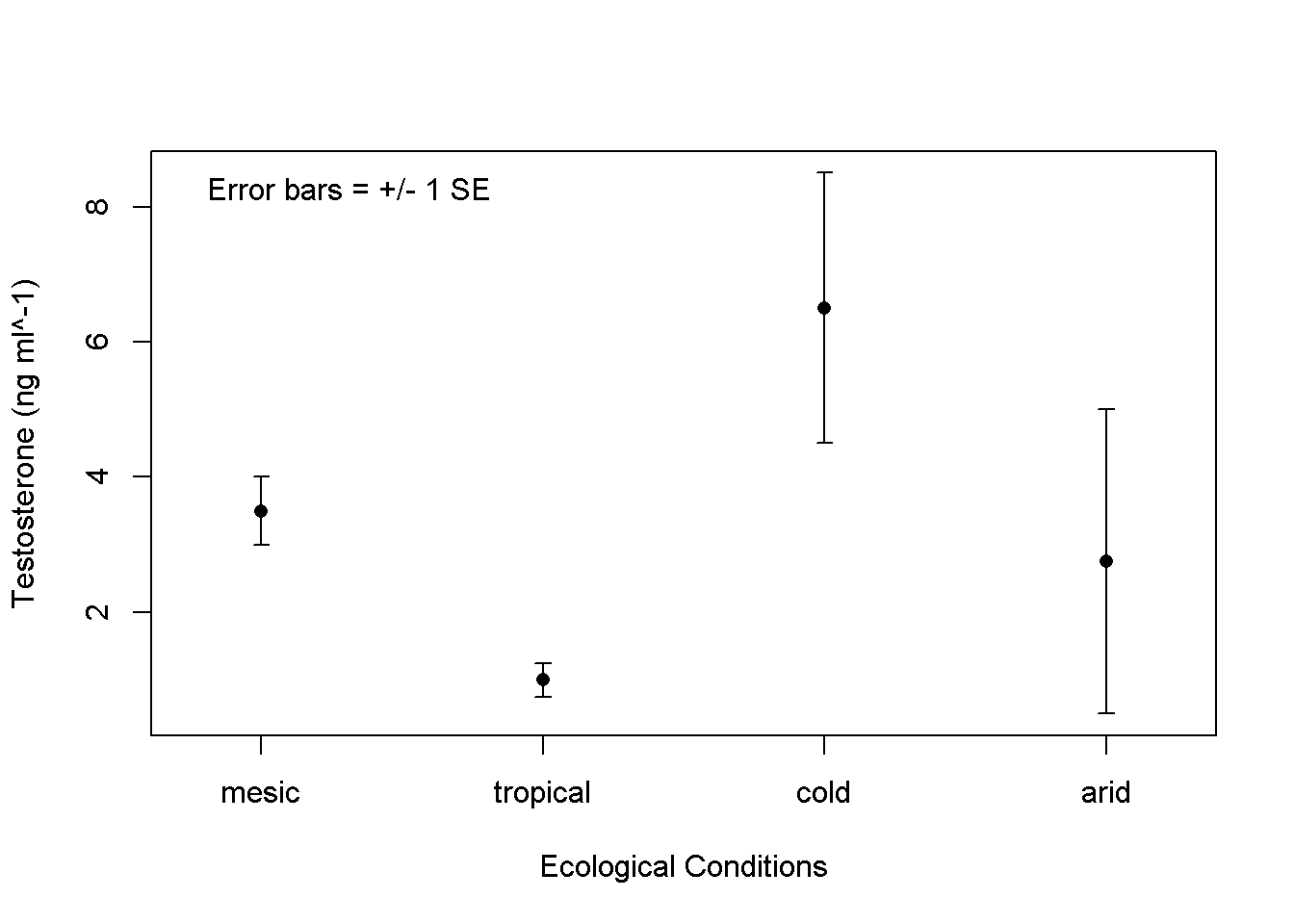The following is a set of annotated code that demonstrates how to make a plot with error bars when you already have the mean values and locations of the error bars for discreate categories of data. The steps are:

• Enter the data into vectors using c()
• Make a simple dataframe using data.frame()
• Plot the data using the errbar() function from the Hmisc() package
• Label the categories along the x-axis using axis()
• Add a notation about what the error bars mean using legend()

The original boxplotThe citation for the paper

Hau et al. 2010. Corticosterone, testosterone and life-history strategies of birds. Proceedings of the Royal Society B. 277:3203-3212

Annotated code to make the graph

#Categories on the x-axis of the study
# these are different ecological condition
# These are all surrounded by " " b/c the are text
category <- c("mesic"
,"tropical"
,"cold"
,"arid")

#These are the mean values represented by bars in the
#original figure
#these are numbers, not text
value.of.point <- c(3.5,1,6.5,2.75)

#Tops of error bars
error.bar.top <- c(4, 1.25, 8.5, 5)

#The length of the error bars
# from the mean to the top of the bar
# This is equal to the standard error (SE)
error.bar.length <- error.bar.top - value.of.point

#Make a dataframe from these values
#  each one has to be separated by a comma
df <- data.frame(category,
value.of.point,
error.bar.top,
error.bar.length)

#THe finished data frame looks like this
#    category value.of.point error.bar.top error.bar.length
# 1    mesic           3.50          4.00             0.50
# 2 tropical           1.00          1.25             0.25
# 3     cold           6.50          8.50             2.00
# 4     arid           2.75          5.00             2.25

#Plot mean values w/error bars using the errbar() function
# from the Hmisc package
# each arguement has to be separated by a comma
# x = c(1,2,3,4) b/c I have 4 categories.
# it would be x = c(1,2,3,4,5) if I have five or
# x = c(1,2,3) if I had 3
# "df" tells errbar() to use data from that dataframe
#  the dollar sign \$ tells errbar() to use the given column
#  xaxt = "n" removes the x axis labels
errbar(x = c(1,2,3,4),   #the number of categories
#along the x axis
y = df\$value.of.point, #mean values to plot
yplus = df\$value.of.point +  #top of error bar
df\$error.bar.length,
ymin = df\$value.of.point -   #bottom of error bar
df\$error.bar.length,
xaxt = "n",          #xaxt removes the numberic lables
xlim = c(0.75,4.25),       #set limit of axes to be +/- 0.25 the number of categories
xlab = "Ecological Conditions",   #label for x-axis
ylab = "Testosterone (ng ml^-1)")  #label for y-axis

# Make Labels for each categories using axis()
#  this adds to an existing plot
axis(side=1,         #1 = the bottom of graph
at=c(1,2,3,4),  #where on x-axis; same as "x" in errbar
labels=df\$category)#what the labels are

#Add an annotation within the graph using legend()
# this adds to an existing plot
legend("topleft", #where to put it; could be "top..." or "bottom...", "...left" or "....right"
legend = "Error bars = +/- 1 SE", #what to write
bty = "n") #remove the box around the legend